# Extensively Evolving Combinator Expressions

S combinator expressions of leaf counts 1 through 13 that do not terminate after 10,000 evolution steps

## Details

The data is calculated using the method shown in the "Scope & additional elements" subsection.
The default content is a Dataset where the rows are labeled by the leaf count of the S combinator expression and contain the combinator expressions that do not terminate after 10,000 evolution steps.

## Examples

### Basic Examples

Get the list of non-terminating S combinator expressions of leaf count 7:

 In:=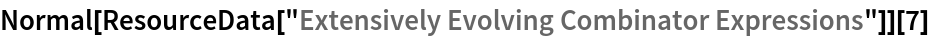Out=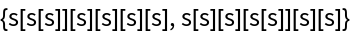Get the length of the list of non-terminating S combinator expressions of leaf count 13:

 In:=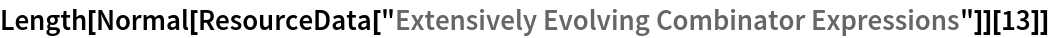Out=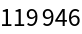Perform from scratch the enumeration of S combinator expressions of leaf count n that do not terminate in max steps. This method can be used to extend the dataset; however, note that as the leaf count for the combinator expressions and maximum steps for the evolutions increase, this computation will become increasingly expensive:

 In:=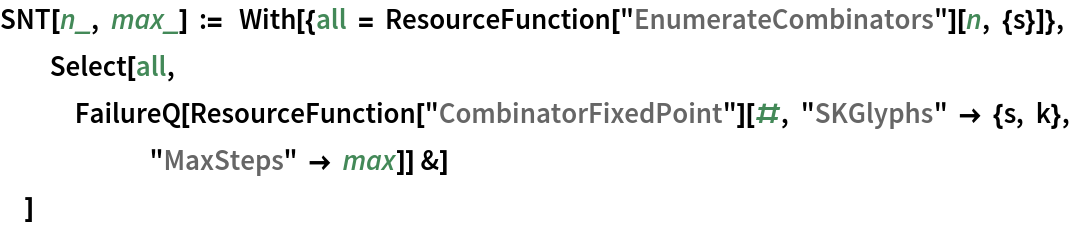Enumerate S combinator expressions of leaf count 8 that do not terminate within 10 steps:

 In:=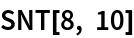Out=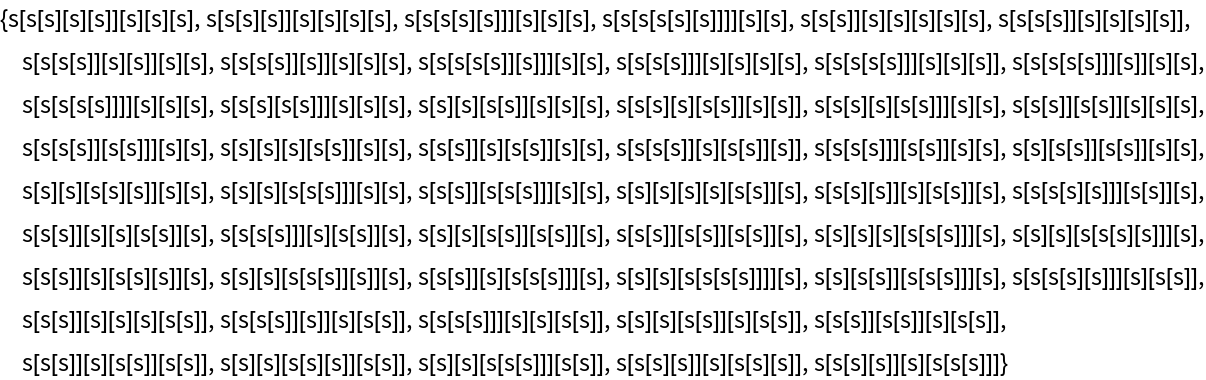Group S combinator expressions of leaf count 7 that terminate within 10,000 steps in equivalence groups designated by their fixed point form:

 In:=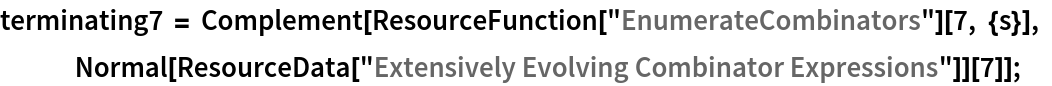In:=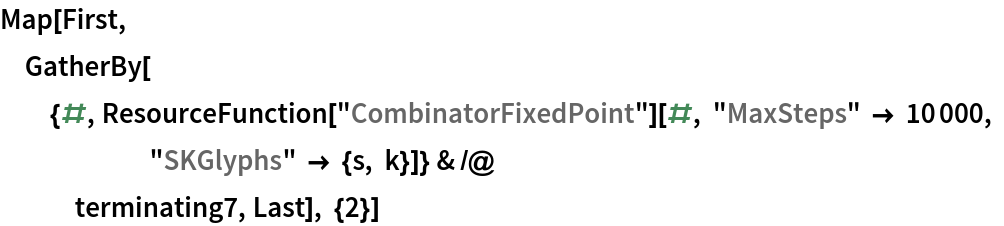Out=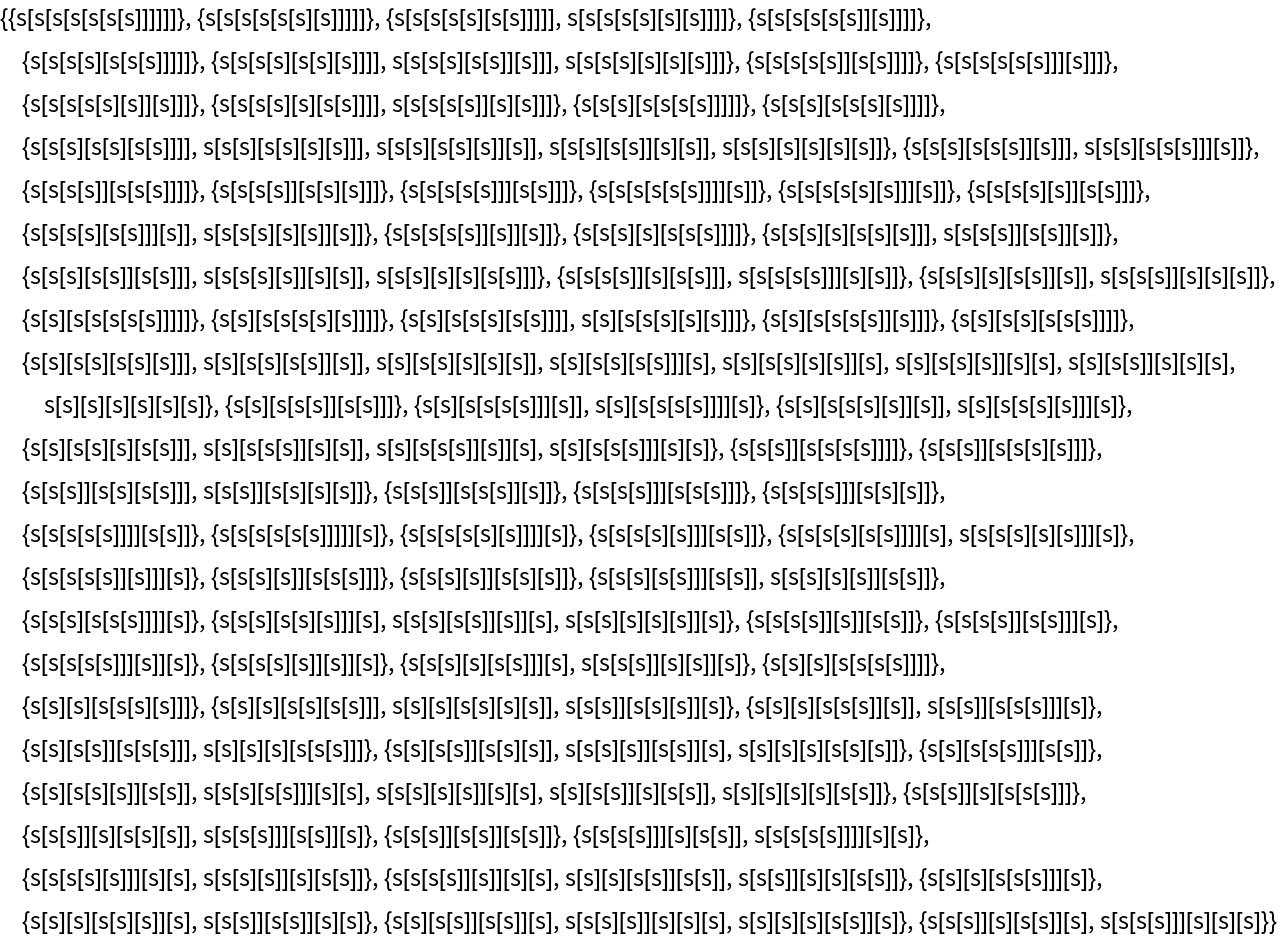Find the distribution of fixed point leaf counts for S combinator expressions of leaf count 7 that terminate within 10,000 steps:

 In:=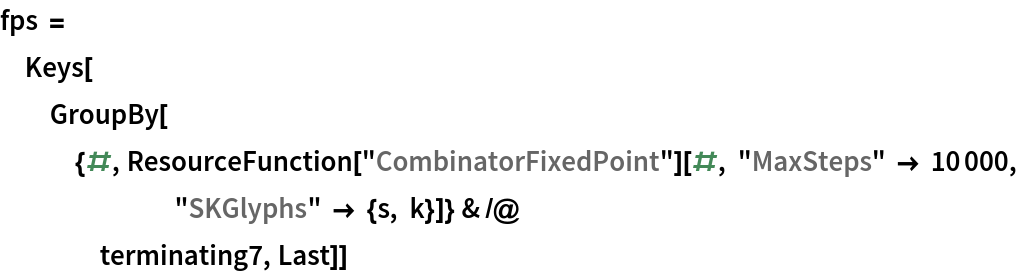Out=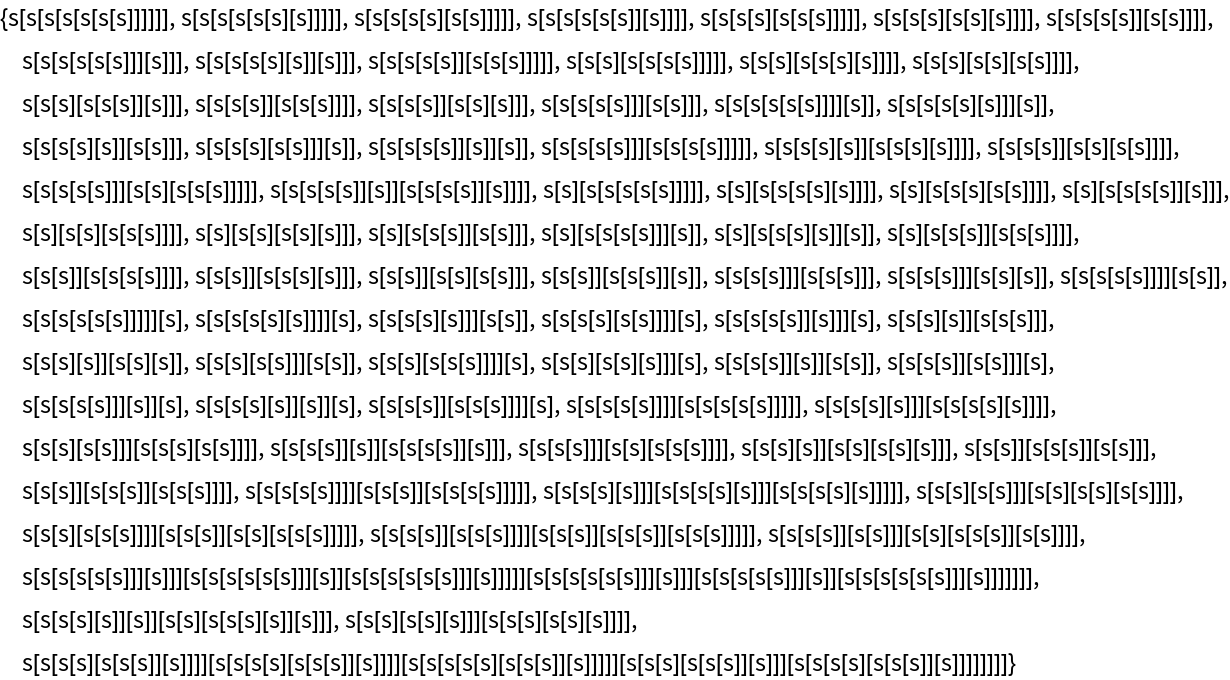In:=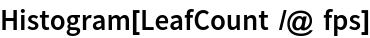Out=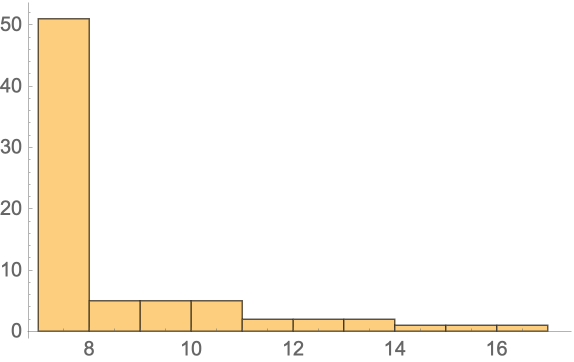Wolfram Research, "Extensively Evolving Combinator Expressions" from the Wolfram Data Repository (2021)